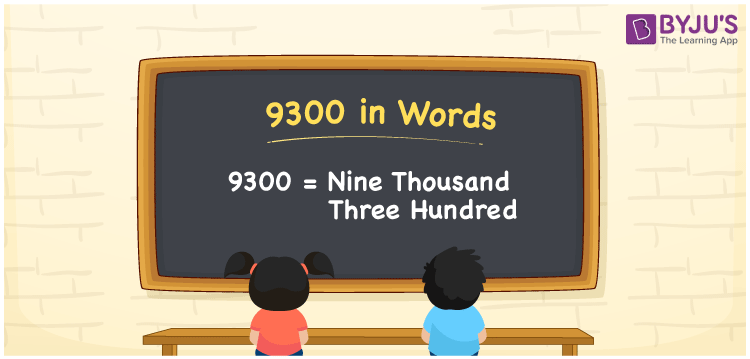# 9300 in words

9300 in words is written as Nine Thousand Three Hundred. 9300 represents the count or value. The article on Counting Numbers can give you an idea about count or counting. The number 9300 is used in expressions that relate to money, distance, length and others. Let us consider an example for 9300. “Adam Gilchrist from Australia, has scored more than Nine Thousand Three Hundred ODI runs.” Another example is, “Last month, Bangalore recorded more than Nine Thousand Three Hundred new Covid cases.”

 9300 in words Nine Thousand Three Hundred Nine Thousand Three Hundred in Numbers 9300

## 9300 in English Words## How to Write 9300 in Words?

We can convert 9300 to words using a place value chart. The number 9300 has 4 digits, so let’s make a chart that shows the place value up to 4 digits.

 Thousands Hundreds Tens Ones 9 3 0 0

Thus, we can write the expanded form as:

9 × Thousand + 3 × Hundred + 0 × Ten + 0 × One

= 9 × 1000 + 3 × 100 + 0 × 10 + 0 × 1

= 9300

= Nine Thousand Three Hundred.

9300 is the natural number that is succeeded by 9299 and preceded by 9301.

9300 in words – Nine Thousand Three Hundred.

Is 9300 an odd number? – No.

Is 9300 an even number? – Yes.

Is 9300 a perfect square number? – No.

Is 9300 a perfect cube number? – No.

Is 9300 a prime number? – No.

Is 9300 a composite number? – Yes.

## Solved Example

1. Write the number 9300 in expanded form

Solution: 9 × 1000 + 3 × 100 + 0 × 10 + 0 × 1

We can write 9300 = 9000 + 300 + 0 + 0

= 9 × 1000 + 3 × 100 + 0 × 10 + 0 × 1

## Frequently Asked Questions on 9300 in words

### How to write 9300 in words?

9300 in words is written as Nine Thousand Three Hundred.

### Is 9300 divisible by 2?

Yes. 9300 is divisible by 2.

### Is 9300 divisible by 10?

Yes. 9300 is divisible by 10.